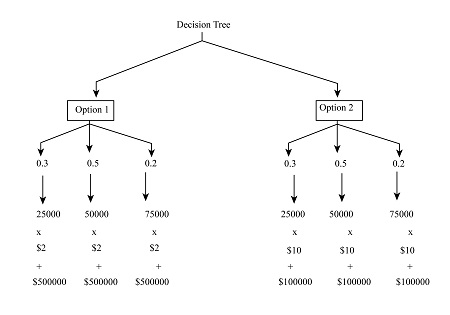# Consider the two capacity options for Arktec Manufacturing show below: Suppose the company has...

## Question:

Consider the two capacity options for Arktec Manufacturing show below:

Fixed cost (per year) Variable cost (per unit)
Option 1 500,000 2
Option 2 100,000 10

Suppose the company has identified the following three possible demand scenarios:

Demand (units per year) Probability
25,000 30%
50,000 50%
75,000 20%

a. Draw a decision tree for Arktec Manufacturing. When drawing your tree, assume that managers must select a capacity option before they know what the demand level will actually be.

b. Calculate the expected value for each decision branch. Which option would you prefer? why?

c. Suppose the lowest and highest demand levels are updated to 40,000 and 110,000 respectively. Recalculate the expected values. Which option would you prefer now?

## Decision Tree

Decision Tree refers to the diagrammatical representation of the given alternative for the company. The alternative are plotted with the given variable with the help of arrows, lines and boxes.

a. Draw a decision tree for Arktec Manufacturing. When drawing your tree, assume that managers must select a capacity option before they know what the demand level will actually be.

The below diagram of decision tree showing both the options with their respective probability. The demand of each option is also shown.b. Calculate the expected value for each decision branch. Which option would you prefer? why?

Option 1:

{eq}\begin{align*} C\,(25000) &= \$500000 + \$ 2 \times 25000\\ &= \$550000\\ C\,(50000) &= \$ 500000 + \$2 \times 50000\\ &= \$ 600000\\ C\,(75000) &= \$500000 + \$ 2 \times 75000\\ &= \650000 \end{align*} {/eq} {eq}\begin{align*} EV(1) &= (\ 550000 \times 30\% ) + (\$600000 \times 50\% ) + (\$ 650000 \times 20\% )\\ &= \$165000 + \$ 300000 + \$130000\\ &= \$ 595000 \end{align*} {/eq}

Option 2:

{eq}\begin{align*} C\,(25000) &= \$100000 + \$ 10 \times 25000\\ &= \$350000\\ C\,(50000) &= \$ 100000 + \$10 \times 50000\\ &= \$ 600000\\ C\,(75000) &= \$100000 + \$ 10 \times 75000\\ &= \850000 \end{align*} {/eq} {eq}\begin{align*} EV(2) &= (\ 150000 \times 30\% ) + (\$600000 \times 50\% ) + (\$ 850000 \times 20\% )\\ & = \$45000 + \$ 300000 + \$170000\\ &= \$ 515000 \end{align*} {/eq}

The expected value of option 2 is less and it should be selected as it is saving good amount of money.

c. Suppose the lowest and highest demand levels are updated to 40,000 and 110,000 respectively. Recalculate the expected values. Which option would you prefer now?

{eq}\begin{align*} C\,(40000) &= \$500000 + \$ 2 \times 40000\\ &= \$580000\\ C\,(50000) &= \$ 500000 + \$2 \times 50000\\ &= \$ 600000\\ C\,(110000) &= \$500000 + \$ 2 \times 100000\\ &= \720000 \end{align*} {/eq} {eq}\begin{align*} EV(1) &= (\ 580000 \times 30\% ) + (\$600000 \times 50\% ) + (\$ 720000 \times 20\% )\\ & = \$174000 + \$ 300000 + \$1440000\\ &= \$ 618000 \end{align*} {/eq}

{eq}\begin{align*} C\,(40000) &= \$100000 + \$ 10 \times 40000\\ &= \$500000\\ C\,(50000) &= \$ 100000 + \$10 \times 50000\\ &= \$ 600000\\ C\,(110000) &= \$100000 + \$ 10 \times 110000\\ &= \1200000 \end{align*} {/eq} {eq}\begin{align*} EV(2) &= (\ 500000 \times 30\% ) + (\$600000 \times 50\% ) + (\$ 1200000 \times 20\% )\\ &= \$150000 + \$ 300000 + \$240000\\ &= \$ 690000 \end{align*} {/eq}

Again, the expected value of option 1 is less and it should be selected as it is saving good amount of money.